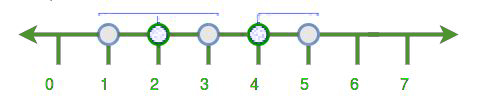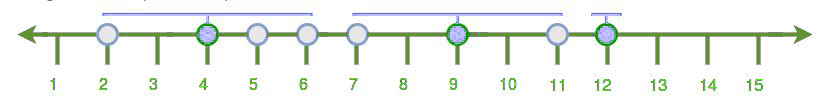# Minimum number of towers required such that every house is in the range of at least one tower

Given a map of the city and the network range, the task is to determine the minimum number of the tower so that every house is within range of at least one tower. Each tower must be installed on top of an existing house.

Examples:

```Input: range : 1
house : 1 2 3 4 5
Output: 2

Input: range : 2
house : 7 2 4 6 5 9 12 11
Output: 3
```

## Recommended: Please try your approach on {IDE} first, before moving on to the solution.All cities can be covered by inserting 2 towers i.e. at house 2 and 4.All cities can be covered by inserting 3 towers i.e. at house 4, 9 and 12.

Algorithm:-

1. First, sort all the element.
2. Count only once and then traverse till its middle house.
3. After this again traverse till tower range.
4. Again repeat 1, 2, 3 step till all the houses are covered.

Below is the implementation of above approach:

## C++

 `// C++ implementation of above approach ` `#include ` `using` `namespace` `std; ` ` `  `// Function to count the number of tower ` `int` `number_of_tower(``int` `house[], ``int` `range, ``int` `n) ` `{ ` ` `  `    ``// first we sort the house numbers ` `    ``sort(house, house + n); ` ` `  `    ``// for count number of twoers ` `    ``int` `numOfTower = 0; ` ` `  `    ``// for iterate all houses ` `    ``int` `i = 0; ` `    ``while` `(i < n) { ` ` `  `        ``// count number of towers ` `        ``numOfTower++; ` ` `  `        ``// find find the middle location ` `        ``int` `loc = house[i] + range; ` ` `  `        ``// traverse till middle location ` `        ``while` `(i < n && house[i] <= loc) ` `            ``i++; ` ` `  `        ``// this is point to middle ` `        ``// house where we insert the tower ` `        ``--i; ` ` `  `        ``// now find the last location ` `        ``loc = house[i] + range; ` ` `  `        ``// traverse till last house of the range ` `        ``while` `(i < n && house[i] <= loc) ` `            ``i++; ` `    ``} ` ` `  `    ``// return the number of tower ` `    ``return` `numOfTower; ` `} ` ` `  `// Driver code ` `int` `main() ` `{ ` `    ``// given elements ` `    ``int` `house[] = { 7, 2, 4, 6, 5, 9, 12, 11 }; ` `    ``int` `range = 2; ` `    ``int` `n = ``sizeof``(house) / ``sizeof``(house); ` ` `  `    ``// print number of towers ` `    ``cout << number_of_tower(house, range, n); ` `} `

## Java

 `// Java implementation of above approach ` ` `  `import` `java.util.Arrays; ` ` `  `public` `class` `Improve { ` ` `  `    ``// Function to count the number of tower ` `    ``static` `int` `number_of_tower(``int` `house[], ``int` `range, ``int` `n) ` `    ``{ ` ` `  `        ``// first we sort the house numbers ` `        ``Arrays.sort(house); ` ` `  `        ``// for count number of towers ` `        ``int` `numOfTower = ``0``; ` ` `  `        ``// for iterate all houses ` `        ``int` `i = ``0``; ` `        ``while` `(i < n) { ` ` `  `            ``// count number of towers ` `            ``numOfTower++; ` ` `  `            ``// find find the middle location ` `            ``int` `loc = house[i] + range; ` ` `  `            ``// traverse till middle location ` `            ``while` `(i < n && house[i] <= loc) ` `                ``i++; ` ` `  `            ``// this is point to middle ` `            ``// house where we insert the tower ` `            ``--i; ` ` `  `            ``// now find the last location ` `            ``loc = house[i] + range; ` ` `  `            ``// traverse till last house of the range ` `            ``while` `(i < n && house[i] <= loc) ` `                ``i++; ` `        ``} ` ` `  `        ``// return the number of tower ` `        ``return` `numOfTower; ` `    ``} ` ` `  ` `  `     `  `    ``public` `static` `void` `main(String args[]) ` `    ``{ ` `        ``// given elements ` `        ``int` `house[] = { ``7``, ``2``, ``4``, ``6``, ``5``, ``9``, ``12``, ``11` `}; ` `        ``int` `range = ``2``; ` `        ``int` `n = house.length; ` ` `  `        ``// print number of towers ` `        ``System.out.println(number_of_tower(house, range, n)); ` ` `  `         `  ` `  `    ``} ` `    ``// This code is contributed by ANKITRAI1 ` `} ` ` `

## Python 3

 `# Python 3 implementation of  ` `# above approach ` ` `  `# Function to count the ` `# number of tower ` `def` `number_of_tower(house, r, n): ` ` `  `    ``# first we sort the house numbers ` `    ``house.sort() ` ` `  `    ``# for count number of twoers ` `    ``numOfTower ``=` `0` ` `  `    ``# for iterate all houses ` `    ``i ``=` `0` `    ``while` `(i < n) : ` ` `  `        ``# count number of towers ` `        ``numOfTower ``+``=` `1` ` `  `        ``# find find the middle location ` `        ``loc ``=` `house[i] ``+` `r ` ` `  `        ``# traverse till middle location ` `        ``while` `(i < n ``and` `house[i] <``=` `loc): ` `            ``i ``+``=` `1` ` `  `        ``# this is point to middle ` `        ``# house where we insert the tower ` `        ``i ``-``=` `1` ` `  `        ``# now find the last location ` `        ``loc ``=` `house[i] ``+` `r ` ` `  `        ``# traverse till last house  ` `        ``# of the range ` `        ``while` `(i < n ``and` `house[i] <``=` `loc): ` `            ``i ``+``=` `1` ` `  `    ``# return the number of tower ` `    ``return` `numOfTower ` ` `  `# Driver code ` `if` `__name__ ``=``=` `"__main__"``: ` `     `  `    ``# given elements ` `    ``house ``=` `[ ``7``, ``2``, ``4``, ``6``, ``5``, ``9``, ``12``, ``11` `] ` `    ``r ``=` `2` `    ``n ``=` `len``(house) ` ` `  `    ``# print number of towers ` `    ``print``(number_of_tower(house, r, n)) ` ` `  `# This code is contributed ` `# by ChitraNayal `

## C#

 `// C# implementation of above approach ` ` `  `using` `System; ` ` `  `public` `class` `Improve { ` ` `  `    ``// Function to count the number of tower ` `    ``static` `int` `number_of_tower(``int` `[]house, ``int` `range, ``int` `n) ` `    ``{ ` ` `  `        ``// first we sort the house numbers ` `        ``Array.Sort(house); ` ` `  `        ``// for count number of towers ` `        ``int` `numOfTower = 0; ` ` `  `        ``// for iterate all houses ` `        ``int` `i = 0; ` `        ``while` `(i < n) { ` ` `  `            ``// count number of towers ` `            ``numOfTower++; ` ` `  `            ``// find find the middle location ` `            ``int` `loc = house[i] + range; ` ` `  `            ``// traverse till middle location ` `            ``while` `(i < n && house[i] <= loc) ` `                ``i++; ` ` `  `            ``// this is point to middle ` `            ``// house where we insert the tower ` `            ``--i; ` ` `  `            ``// now find the last location ` `            ``loc = house[i] + range; ` ` `  `            ``// traverse till last house of the range ` `            ``while` `(i < n && house[i] <= loc) ` `                ``i++; ` `        ``} ` ` `  `        ``// return the number of tower ` `        ``return` `numOfTower; ` `    ``} ` ` `  ` `  `     `  `    ``public` `static` `void` `Main() ` `    ``{ ` `        ``// given elements ` `        ``int` `[]house = { 7, 2, 4, 6, 5, 9, 12, 11 }; ` `        ``int` `range = 2; ` `        ``int` `n = house.Length; ` ` `  `        ``// print number of towers ` `        ``Console.WriteLine(number_of_tower(house, range, n)); ` ` `  `         `  ` ``// This code is contributed by inder_verma.. ` `    ``} ` `     `  `} `

## PHP

 ` `

Output:

```3
```

Time Complexity: O(nlogn)
Space Complextity: O(1)

My Personal Notes arrow_drop_upCheck out this Author's contributed articles.

If you like GeeksforGeeks and would like to contribute, you can also write an article using contribute.geeksforgeeks.org or mail your article to contribute@geeksforgeeks.org. See your article appearing on the GeeksforGeeks main page and help other Geeks.

Please Improve this article if you find anything incorrect by clicking on the "Improve Article" button below.